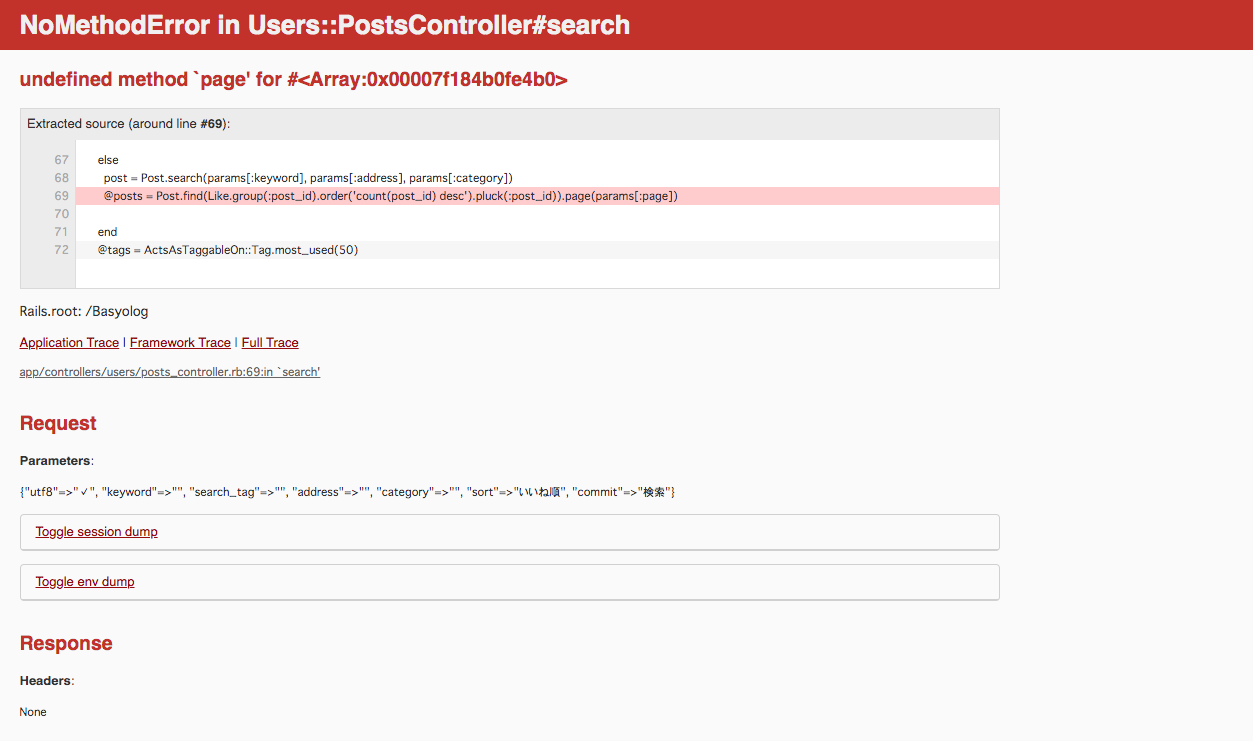# [Ruby] How to implement ranking function with Rails

Previously, I implemented the ranking function by referring to this article of Qiita (Rapid ranking function) was. I was able to implement it safely, but I faced the following problems.

It doesn’t work when combined with the pagination feature of # kaminari!

``````@posts = Post.find(Like.group(:post_id).order('count(post_id) desc').pluck(:post_id)).page(params[:page])
``````

When combined with kaminari’s page method, the result is as above, but with this, the following error occurs.Therefore, we will introduce a ranking function that does not have a problem even when combined with the page method.

# Premise

• Article table name is posts
• Like table names are likes
• You have already implemented the Like function and have an association between posts and likes

# Implementation of ranking function

``````@posts = Post.joins(:likes).group(:post_id).order('count(post_id) desc')
``````

This is OK! I will explain one by one.

``````Post.joins(:likes) Inner join #posts table and likes table
group(:post_id) # divide the group into those with the same post_id
order('count(post_id) desc') # sort it in order of most post_id
``````

This completes the ranking function. As shown below, even if the page method is added to the end of the code, no error occurs and I think the pagination function is working.

``````@posts = Post.joins(:likes).group(:post_id).order('count(post_id) desc').page(params[:page])
``````
• Easy ranking function with Rails
https://qiita.com/mitsumitsu1128/items/18fa5e49a27e727f00b4